# 基于投影寻踪模拟退火的多传感信息融合技术Multi-Sensor Information Fusion Technology Based on Projection Pursuit Simulated Annealing

DOI: 10.12677/JSTA.2020.82002, PDF, HTML, XML, 下载: 245  浏览: 655  国家自然科学基金支持

Abstract: Based on the advantages of weighted average method and probability statistics method, a multi-sensor information fusion algorithm based on projection pursuit simulated annealing method is designed in this paper. The innovation of the design lies in the addition of probability window constraint and clustering constraint in the process of projection weight adjustment to reduce the computational complexity of the system by 41.6%, and the results obtained are in line with the peak regression judgment. This design has a new improvement to solve the multi-sensor information fusion of the system, and has a certain improvement to the real-time and applicable range.

1. 绪论

5G时代，万物互联不再是空话。高达TB级的传输速率和海量节点数，为智能生产生活赋予了强大的助推力。未来，空间里将覆盖着密集的传感器网络，对信息的把控将更为彻底。许多情况下，并不需要了解每一个传感器的数据，更想获取的是区域内的总体指标参数，简化决策，实时找到故障区域。为解决这一问题，多传感器的信息融合技术正在被众多学者研究 。但其相关技术、算法，都有一定的局限性和缺陷。所以，还需要进一步的探索，为5G时代传感器的改革创新铺设通畅的道路。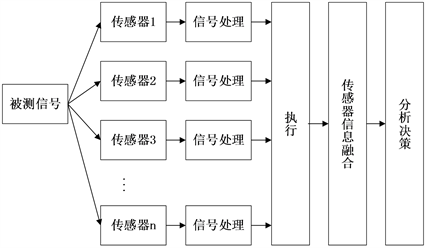Figure 1. Multi-sensor information fusion process

(1) 数据固有缺陷：不和谐数据奇点、偶然误码。

(2) 量纲和维度：量纲、维数不统一。

(3) 数据关联：正负性指标需分别处理、数据类型多。

2. 方法

2.1. 前人的方法及改进流程

$\left({m}_{1}\oplus {m}_{n}\oplus \cdots \oplus {m}_{n}\right)\left(A\right)=\frac{1}{1-k}\underset{A1\cap A2\cap \cdots \cap An=A}{\sum }{m}_{1}\left(A1\right){m}_{1}\left(A1\right)\cdots {m}_{1}\left(An\right)$

(2) 加权平均法，加权平均法基本原理是观察大量样本，取平均权值作为经验权值，将各个样本数值与权值进行累积求和。基本公式为 ${s}_{j}={\sum }_{i=1}^{n}{c}_{ij}{w}_{ij}$，其中 ${w}_{ij}$ 为样本j的第i个指标的经验权值， ${c}_{ij}$ 为第j个样本值。(3)概率论方法，概率论法是基于频率和权数的转化方法，在加权平均法基础上进一步作出的改进。研究的思想是某一类信息数据对系统出现较大影响频率作为该类型信息的权重划分依据。基本公式如下：

$\stackrel{^}{{\mu }_{i}}=\frac{1}{m}\underset{j=1}{\overset{n}{\sum }}{x}_{ij}$

${\stackrel{^}{{\sigma }_{i}}}^{2}=\frac{1}{m-1}\underset{j=1}{\overset{n}{\sum }}\left({x}_{ij}-{\stackrel{^}{{\mu }_{i}}}^{2}\right)$

2.2. 统一量纲化及正负性指标处理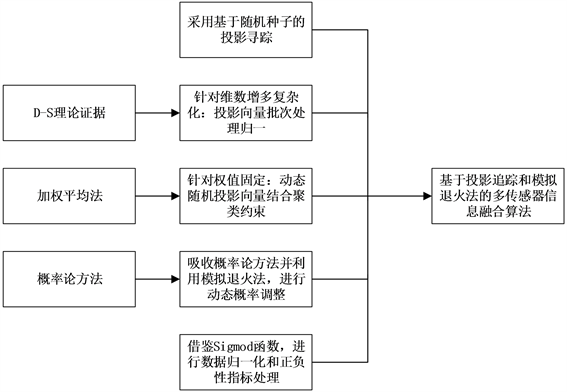Figure 2. Multi-sensor information fusion algorithm to improve the process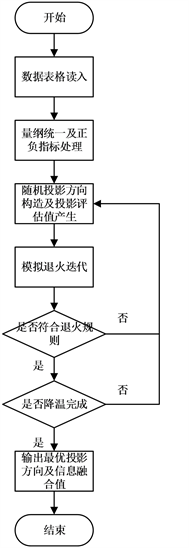Figure 3. Overall flow chart of fusion algorithm

${x}_{j}=\frac{{x}_{j}-\mathrm{min}{x}_{j}}{\mathrm{max}{x}_{j}-\mathrm{min}{x}_{j}}$

${x}_{j}=\frac{\mathrm{max}{x}_{j}-{x}_{j}}{\mathrm{max}{x}_{j}-\mathrm{min}{x}_{j}}$

2.3. 投影寻踪法原理及应用

2.3.1. 投影方向的设计

${z}_{j}=\underset{j=1}{\overset{m}{\sum }}{a}_{j}{x}_{ij}$

$\left\{\begin{array}{l}{a}_{j}>0\\ \underset{j=1}{\overset{m}{\sum }}{a}_{ij}^{2}=1\end{array}$

2.3.2. 投影方向优良评估设计

$\mathrm{max}Q\left(a\right)={S}_{a}{D}_{a}$

2.4. 模拟退火法原理及应用

2.4.1. 模拟退火法的原理

${P}_{ij}^{T}=\left\{\begin{array}{l}1,E\left(j\right)\le E\left(i\right)\\ {\text{e}}^{-\left(\frac{E\left(j\right)-E\left(i\right)}{KT}\right)},其他\end{array}$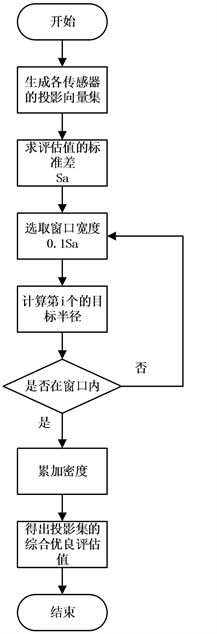Figure 4. Projection direction good evaluation flowchart

2.4.2. 模拟退火法在本文中的应用

2.4.3. 模拟退火法流程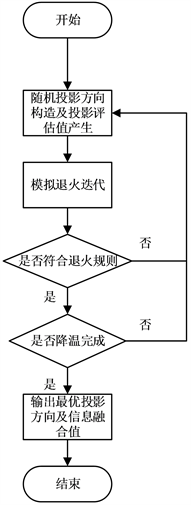Figure 5. Simulated annealing process

3. 结果

Table 1. Partial sensor data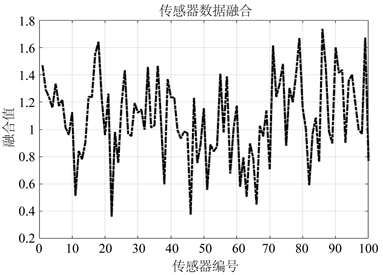Figure 6. Sample comprehensive evaluation value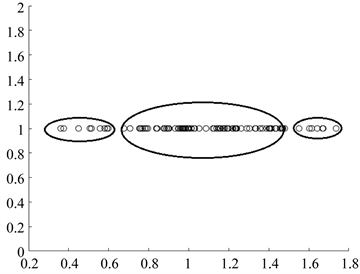Figure 7. Sample comprehensive evaluation scatter distribution

86号样本数据：

22号样本数据：

42号样本数据：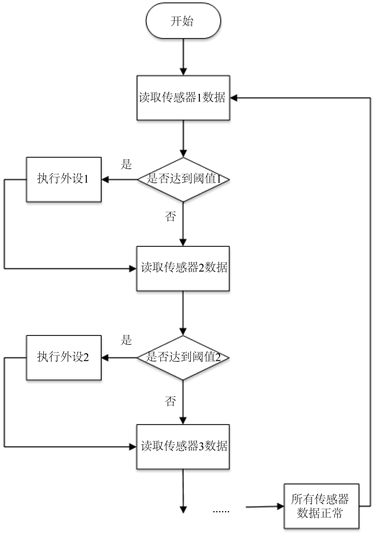Figure 8. Traditional non-fusion single sensor data acquisition and decision-making mode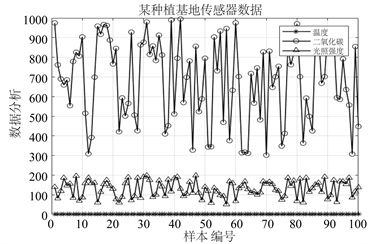Figure 9. Data graph of multiple sensors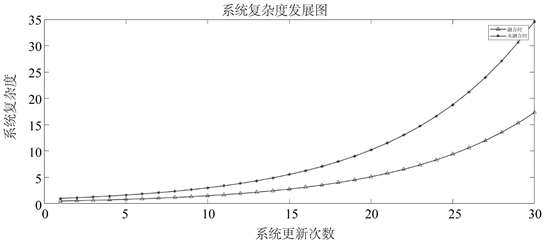Figure 10. The development chart of system complexity using fusion technology and non- fusion technology

4. 结论与讨论

5G时代，万物互联。多传感器信息融合技术给空间传感器网络带来智能化的监控管理。对于多传感器信息融合技术的算法研究将持续进行。随着智能传感技术的不断发展和融合技术的不断完善，人们对于系统或者设备的整体状态的把握将更加精确。智能生活，万物互联的未来已然来临。

NOTES

*通讯作者。

  赵宗贵. 信息融合技术现状、概念与结构模型[J]. 中国电子科学研究院学报, 2006(4): 305-312.  Zhou, Y.H., Zuo, Z.T. and Xu, F.R. (2019) Origin Identification of Panax Notoginseng by Multi-Sensor Information Fusion Strategy of Infrared Spectra Combined with Random Forest. Spectrochimica Acta-Part A: Molecular and Biomolecular Spectroscopy, Vol. 226, 5 February 2019. https://doi.org/10.1016/j.saa.2019.117619  刘国凯. 基于多传感信息融合的旋转机械故障诊断研究[D]: [硕士学位论文]. 贵阳: 贵州大学, 2018.  赵毅. 数字滤波的算术平均法和加权平均法[J]. 仪表技术, 2001(4): 41-44.  周治利. 基于概率假设密度的多传感器多目标跟踪技术研究[D]: [硕士学位论文]. 杭州: 杭州电子科技大学, 2017.  Šárka, B., Peter, F., Thomas, O., Christian, B. and Maia, R. (2019) Robust and Sparse K-Means Clustering for High Dimensional Data.  张欣莉, 丁晶, 李祚泳, 金菊良. 投影寻踪新算法在水质评价模型中的应用[J]. 中国环境科学, 2000(2): 187-189.  王顺久, 张欣莉, 丁晶, 侯玉. 投影寻踪聚类模型及其应用[J]. 长江科学院院报, 2002(6): 53-55+61.  金菊良, 魏一鸣, 付强, 丁晶. 农业生产力综合评价的投影寻踪模型[J]. 农业系统科学与综合研究, 2001(4): 241-243.  任恒妮. 大数据K-means聚类算法的研究与应用[J]. 信息技术, 2019, 43(11): 20-23.  饶太春, 兰林强, 罗伟林. 模拟退火法在协同优化中的应用[J]. 福州大学学报(自然科学版), 2018, 46(3): 379-385.